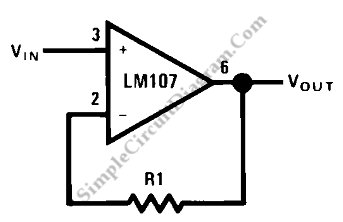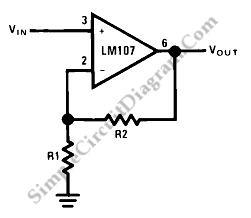## Very Simple Lead Acid Battery Charger with PB137 RegulatorThis is a Very Simple circuit for Lead Acid Battery Charger using PB137 Regulator. The PB137 is used for lead acid battery charger circuit because it can give 1.5A at Vo=13.7V. The PB137 also has a reverse leakage current. The maximum reverse leakage current of PB137 is 10µA at Tj=0 to 40°C and V1=floating and Vo=13.7V. Here is the circuit […]

## Op-Amp Application: Unity Gain BufferThis circuit is an Unity Gain Buffer. This circuit has a highest input impedance of any OP-AMP circuit. This circuit has a less gain error that is equal to common mode rejection or the reciprocal of the amplifier open-loop gain. The multiplication of the open-loop gain and the differential input impedance parallel with common mode input impedance will result the […]

## Op-Amp Application: Non-Inverting AmplifierThis is a high input impedance non-inverting circuit. This circuit provides a closed loop gain equal to (R1+R2)/R1. The close-loop 3 dB bandwidth of this circuit equal to the amplifier unity-gain frequency divided by the closed-loop gain. Here is the circuit : This circuit is different from an inverting circuit. The input impedance of this circuit is very high and […]